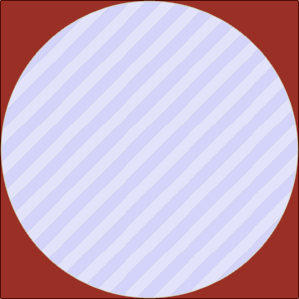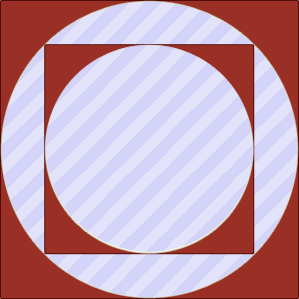### Problem Statement

Draw a square with side length sideLength on a plane. Then, inscribe a circle in the square. Inscribe another square in that circle, and yet another circle in the second square. Continue this process until K circles appear on the plane.

For example, if K=3, the picture would look like this:For each circle, compute the area within the circle that does not belong to any other figure inside that circle. Return the sum of those areas. In the example above, the area to compute is colored in stripes.

### Definition

 Class: RecursiveFigures Method: getArea Parameters: int, int Returns: double Method signature: double getArea(int sideLength, int K) (be sure your method is public)

### Notes

-Your return value must have an absolute or relative error less than 1e-9.
-The area of square with side length A is A*A.
-The area of circle with radius R is pi*R*R.
-The length of diameter of the circle inscribed in a square is equal to the square's side length.
-The side length of the square inscribed in circle with radius R is equal to R*sqrt(2).

### Constraints

-sideLength will be between 1 and 100, inclusive.
-K will be between 1 and 10, inclusive.

### Examples

0)

 `10` `1`
`Returns: 78.53981633974483`1)

 `10` `2`
`Returns: 67.80972450961724`2)

 `10` `3`
`Returns: 62.444678594553444`

#### Problem url:

http://www.topcoder.com/stat?c=problem_statement&pm=10681

#### Problem stats url:

http://www.topcoder.com/tc?module=ProblemDetail&rd=14145&pm=10681

gojira_tc

#### Testers:

PabloGilberto , legakis , ivan_metelsky

#### Problem categories:

Geometry, Simple Search, Iteration